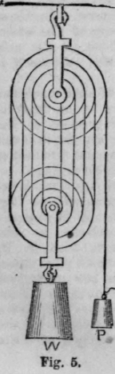Pulleys. The pulley is a wheel, round the rim of which a groove is cut, in which a cord can work, and the centre of which moves on pivots in a block. The wheel sometimes passes under the name of a sheave.

By a fixed pulley, we mean one which merely revolves on its axis, but does not change its place. The power is applied to one end of the cord and the weight to the other.

The action of the pulley may be readily understood from that of the lever. Let c, fig. 1 be the axis of the pulley, b the point to which the weight is attached; a the point of application of the power; draw the Ones, c b,c a - they represent the arms of a lever - and the law of the equilibrium of a lever, therefore, applies in this case also; and as these are necessarily equal to each other, the pulley will be in equilibrio when the weight and power are equal.

If the direction in which the power is applied, instead of being P a, is P a, the same reasoning holds good. For, on drawing C a, as before, it is obvious that b c a represent a bent lever of equal arms. The condition of equilibrium is, therefore, the same.

The fixed pulley does not increase the power, but it renders it more available, by permitting us to apply it in any desired direction.

To prove the properties of the pulley experimentally, hang to the ends of its cord equal weights; they will remain in equi-•librio. Or, if the power be increased, so as to make the weight ascend, the vortical distances passed over are equal.

The moveable pulley is represented at fig. 2. Its peculiarity is that, besides the motion on its own axis, it also has a progressive one. Let b be the axis of the pulley, and to it the weight, W, is attached the power as applied at a. Draw the diameter, a c, then c is the fulcrum of a c, which is in reality a lever of the third order in which distance, a c, of the power is twice that, b c, of the weight. Consequently " the moveable pulley doubles the effect of the power," and the distance traversed by the power is twice that traversed by the weight.A moveable pulley is sometimes called " a runner;" and as it would be often inconvenient to apply the power in the upward direction,as at a, there is commonly associated with the runner a fixed pulley, which without changing the value of the power, enables us to vary the direction of its action. Systems of pulleys are arrangements of sheaves, moveable and fixed. When one fixed pulley acts on a number of moveable ones, equilibrium is maintained, when the power and weight are to each other as 1 to that power of 2 which equals the number of themove-able pulleys. Thus, if there be, as in fig. 3, three moveable pulleys, the power is to the weight as 1:2' that is 1:8; consequently, on such a system, a given power will support an eightfold weight.

When several moveable and fixed pulleys are employed, as in fig. 4, equilibrium is obtained when the power equals the weight divided by twice the number of moveable pulleys. The weight being equally divided between the six lines, it follows that each is drawn by one-sixth of the weight, consequently, if sixty pounds weigh is suspended to the bottom, each line would be drawn upon by a force of 1g pounds. If we wis], to keep this maching in a state of equilibrium, we must attach a weight, P, of ten pounds to the end of the line.In such systems of pulleys there is a great loss of power arising from the friction of the sheaves against the sides of the blocks, and on their axles. In White's pulley this is to a considerable extent a-voided. This contrivance is represented in fig. 5. It consists of several sheaves of unequal diameters, all turned on one common mas3, and working on one common axis. The diameters of these, in the upper blocks, are as the numbers 2, 4, 6, etc, and in the lower 1, 3, 5, etc.; consequently, they all revolve in equal times and the rope passes without sliding or scraping upon the grooves.